Courses

# MCQ (Previous Year Questions) - Isomerism (Level 2)

## 14 Questions MCQ Test Chemistry for JEE Advanced | MCQ (Previous Year Questions) - Isomerism (Level 2)

Description
This mock test of MCQ (Previous Year Questions) - Isomerism (Level 2) for JEE helps you for every JEE entrance exam. This contains 14 Multiple Choice Questions for JEE MCQ (Previous Year Questions) - Isomerism (Level 2) (mcq) to study with solutions a complete question bank. The solved questions answers in this MCQ (Previous Year Questions) - Isomerism (Level 2) quiz give you a good mix of easy questions and tough questions. JEE students definitely take this MCQ (Previous Year Questions) - Isomerism (Level 2) exercise for a better result in the exam. You can find other MCQ (Previous Year Questions) - Isomerism (Level 2) extra questions, long questions & short questions for JEE on EduRev as well by searching above.
QUESTION: 1

### Which of the following compounds will exhibit cis-trans isomerism?

Solution:
There will be 6 isomers possible for this formula.
take two halogens on any one of doubly bonded carbon and u would have three possible combination.
For each of this three structure each one will have cis and trans structures and so total 6.
QUESTION: 2

### Which of the following compounds exhibits stereoisomerism?              [JEE 2002(Scr.)]

Solution:
Since the compounds are optically active, it exhibits stereoisomerism.
HOOC---CH---CH2---CH3
|
CH2
QUESTION: 3

### In the given conformation, if C2 is rotated about C2 - C3 bond anticlockwise by an angle of 120° then the conformational obtained is                  [JEE 2004(Scr.)]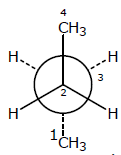Solution: When you rotate 120 degrees of c3 carbon then c1 when the carbon-rich position of C3 carbon atom. then this form is more stable because angle atoms are maximum. this process is known as gauche process angle between two atoms is 60 degree.
QUESTION: 4

The number of structural isomers for C6H14 is                    [JEE 2007]

Solution:
QUESTION: 5

Statement-1: Molecules that are not superimposable on their mirror images are chiral. because

Statement-2: All chiral moleculs have chiral centres.                       [JEE 2007]

Solution:
*Multiple options can be correct
QUESTION: 6

The correct statement(s) about the compuond given below is (are)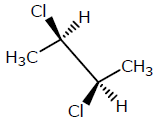Solution: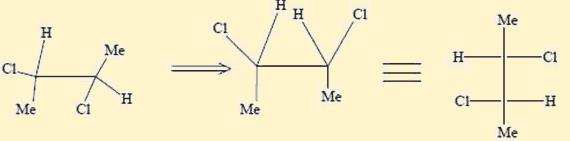The molecule is optically active.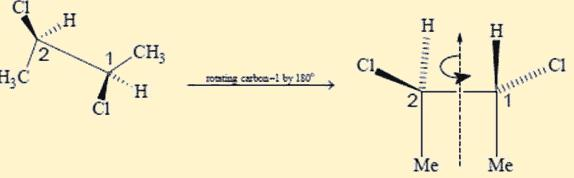The molecule dominate an axis of symmetry (C2) perpendicular to the C – C bond

*Multiple options can be correct
QUESTION: 7

The correct statement(s) concerning the structures E, F and G is (are)           [JEE 2008]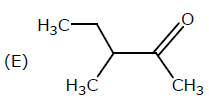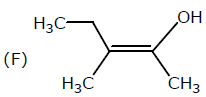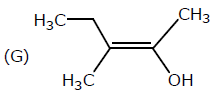Solution:
*Multiple options can be correct
QUESTION: 8

The correct statement(s) about the compound H3C(OH)HC - CH = CH - CH(OH)CH3(X) is (are)

[JEE 2009]

Solution:
*Multiple options can be correct
QUESTION: 9

In the Newman projection for 2,2-dimethylbutane                              [JEE 2010]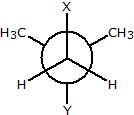X and Y cn respectively be

Solution:
*Multiple options can be correct
QUESTION: 10

Amongst the given options, the compound(s) in which all the atoms are in one plane in all the possible conformations (in any), is (are)                  [JEE 2011]

Solution:
QUESTION: 11

In Allene (C3H4), the type (s) of hybridisation of the carbon atoms is (are)             [JEE 2012]

Solution:
QUESTION: 12

The number of optically active product obtained from the complete ozonolysis of the given compound is

[JEE 2012]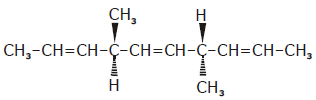Solution:
*Multiple options can be correct
QUESTION: 13

Which of the following molecules in pure from is (are) unstable at room temperature         [JEE 2012]

Solution: (B) Eyclobutadiene follow Huckel's criteria for anti-aromaticity, hence unstable. (C) Follows Huckel's criteria for antiaromaticity, hence, unstable.
*Multiple options can be correct
QUESTION: 14

Which of the given statement(s) about N, O, P and Q with respect to M is (are) correct?     [JEE 2012]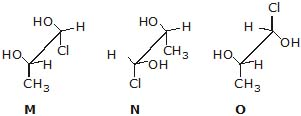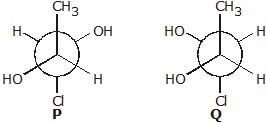Solution:

Fischer Projections of the molecules: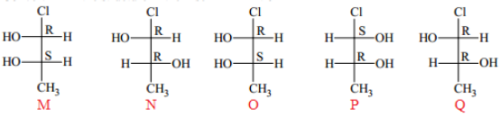It is clear that

M and N are non–mirror image stereoisomers.

M and O are the same molecules i.e. Identical

M and P are the non-superimposable mirror images of hence they are enantiomers.

N and Q are identical

M and Q are also  are non–mirror image stereoisomers i.e  diasteriomers.

Hence A, B and C are the correct statements.